## 9.03.2016

### The linear angular functions

Subject of occupations:
TRIGONOMETRIC FUNCTIONS IN A RECTANGLE
Subject of the previous lesson
Division

Lesson 14

The linear angular functions

If to consider terminating trigonometric functions as coordinates of points of a unit circle in a Cartesian coordinate system, then the linear angular functions are coordinates of points of the chord connecting circle cross points to a coordinate. The sum of coordinates of any point of this chord is always equal to unit.The linear angular functions

In mathematician of a concept, similar to the linear angular functions, are used since ancient times - it is division whole on a part. In the modern world an analog are percent.

The explanation for readers of this website. For myself I called the linear angular functions "linos" and "loses". How I thought up these names? Took designation of a sine and cosine. Visually they quite well differ. In each designation I replaced the first letter with the Latin letter "l" from the word "line". It turned out quite nicely. But to solve to you. Whether these functions in mathematician will get accustomed and as they will be called - time will show. I just offer one more mathematical tool for the description of reality.

At the following lesson we will consider

### Division

Subject of occupations:
TRIGONOMETRIC FUNCTIONS IN A RECTANGLE
Subject of the previous lesson
Decomposition on factors

Lesson 13

Division

Contrary to the standard opinion, division is not mathematical operation. This solution of a standard task of finding of one of factors if other factor and result of multiplication is known. In ancient Babylon the fraction was considered as result of multiplication of number to inverse other number. Even in the modern mathematics there is no division of one fraction into other fraction, this operation is replaced with multiplication of a dividend to fraction, inverse to a divider.

Division can be considered as a projection of result of multiplication along one of factors. For example, length is a projection of the area along width, width is a projection of the area along length.

The most interesting in this plan is the speed which is measured by the lengthiest, divided into time. If to assume that length is result of multiplication of two perpendicular directions of time, then speed is a projection of length (the area of time) along one of the directions of time. For a comprehension of the nature and an essence of light velocity, this approach can be the very useful.

At the following lesson we will consider
The linear angular functions

### Decomposition on factors

Subject of occupations:
TRIGONOMETRIC FUNCTIONS IN A RECTANGLE
Subject of the previous lesson
Zero and infinity

Lesson 12

Decomposition on factors

Mathematical operation, opposite on sense to multiplication, decomposition on factors is. It is carried out with application of the infinite trigonometric functions.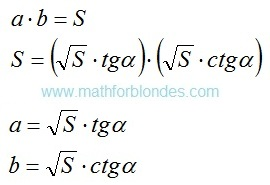Decomposition on factors
The most prime example of decomposition on factors at an angle in 45 degrees is root squaring. As both factors in this case are identical, as result of decomposition it is accepted to write down only one of factors.

Decomposition on factors can be applied when only the result of multiplication is known and any of factors is not known. Units of measure as a result decomposition on factors should be selected intuitively so that as a result of their multiplication the tentative unit of measure turned out. The quantity of spacelike dimensions in units of measure of factors at decomposition can be a miscellaneous. For example, three-dimensional volume can be spread out to one-dimensional factors by means of two operations of decomposition, one of options looks so:Decomposition of volume

In this example corners α and β are not bound among themselves. If to display volume in a cube (a=b=c), then α≈35° is a angle between the diagonal of a cube and diagonal of the basis, β=45 ° is a angle between the diagonal of the basis and its party.

At the following lesson we will consider
Division

## 8.19.2016

### Zero and infinity

Subject of occupations:
TRIGONOMETRIC FUNCTIONS IN A RECTANGLE
Subject of the previous lesson

Lesson 11

Zero and infinity

If the angle is equal to zero or 90°, then the two dimensional rectangle disappears and there is a one-dimensional piece. From here the sense of infinity follows: as if we did not change the party of a rectangle, it will never turn into a piece. Unit divided into zero is not equal to infinity. Infinitesimal size is not equal to unit divided into infinity.Zero and infinity

Difference between elements in these inequalities same as difference between the point lying on a straight line and the point which is not lying on a straight line.

Multiplication to zero and division by zero do not fall into to mathematical operations with numbers, they are carried out in the field of units of measure. It is possible to call these values of trigonometric functions non-numerical.

In addition to the materials about multiplication and division by zero explained earlier it is necessary to add the following. In positional notation zero designates lack of number of the particular category. Lack of number number cannot be. Here zero is similar to punctuation marks in writing which have the graphic form, but are not said when reading.

Generally zero should be understood as lack of the considered unit of measure. For example, zero value of a angle means that the angle is absent. Division by zero should be considered as need of introduction of a unit of measure, perpendicular to already existing, for the further solution of a task. Division by zero does not mean automatic transition to multiplication. For example, it is impossible to describe turn of a piece in one-dimensional space, for this purpose it is necessary to enter padding measurement and to consider a task in two dimensional space.

At the following lesson we will consider
Decomposition on factors

## 8.14.2016

### Distinctions between multiplication and addition

Subject of occupations:
TRIGONOMETRIC FUNCTIONS IN A RECTANGLE
Subject of the previous lesson
Examples of multiplication

Lesson 10

Multiplication is a change of quality, that is change of units of measure. Contrary to the standard opinion, multiplication cannot be presented in the form of addition. When multiplication is substituted for addition, mathematical properties of multiplication are used. With units of measure substitution looks so: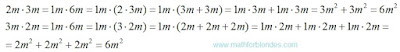Multiplication substitution by addition

Algebraic expressions with use of letters show distinction between addition and multiplication:Multiplication in algebra

If at addition items have identical numerical values, then it is possible to apply mathematical model of multiplication for addition. At the same time we assume that units of measure at multiplication do not change: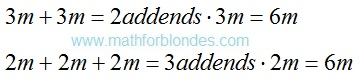Addition replacement with multiplication

In mathematician possess separate properties of units of measure:

- number systems of numbers – it is impossible to addition the numbers presented in different number systems, a question of a possibility of multiplication of numbers in different number systems the author it was not studied;

- denominators of common fractions – it is impossible to addition fractions with different denominators, at multiplication of fractions denominators are multiplied;

- alphabetic references in algebraic expressions – it is impossible to addition numbers with different designations, at multiplication new designation of result turns out;

- legends of functions (for example, trigonometrical).

At the following lesson we will consider
Zero and infinity

### Examples of multiplication

Subject of occupations:
TRIGONOMETRIC FUNCTIONS IN A RECTANGLE
Subject of the previous lesson
Multiplication

Lesson 9

Examples of multiplication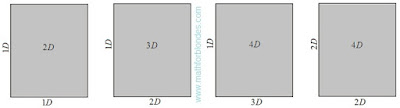Multiplication in space

At interaction of two one-dimensional spaces (in figure D is quantity of spacelike dimensions) the two dimensional space turns out. At addition to interaction of perpendicular measurement, the quantity of spacelike dimensions of result increases. It is necessary to understand any quantities participating in multiplication as spacelike dimensions. Multiplication of two one-dimensional lengths gives the two dimensional area, multiplication of the two dimensional area at one-dimensional length gives three-dimensional volume and so on. The possibility of receiving four-dimensional space as a result of multiplication of two two dimensional spaces is theoretically possible, but demands specification from the point of view of physical reality.Examples of multiplication

Voltage is result of interaction of current intensity and resistance. If to consider current intensity not as one-dimensional quantity and as two dimensional (the area of current), then as a result of interaction with a resistance power will turn out. Units of measure of the specified quantities (Volts, Ampere, Ohms, Watts) are household (convenient in daily use), but demand mathematical representation for comprehension of an essence of the physical processes expressed by these units of measure. The system of physical quantities offered R.O. di Bartini can be considered as attempt of transition from household units of measure to mathematical. Interaction between quantities is carried out due to the processes studied in physics.

The arrangement of threads in the perpendicular direction allows to receive cloth. Interaction between threads is carried out at the expense of frictional force. If in the perpendicular directions to arrange reinforcing rod stock, then it is possible to receive a two dimensional reinforcing grid or a three-dimensional reinforcing framework. Interaction of separate rod stock in clusters is provided with electric welding or a knitting wire. Plywood is the fibers of a wood neveer located perpendicularly. Interaction is provided by pasting of separate layers of wood neveer.

At multiplication the quantity of goods on unit price, as a result turns out the cost of a consignment of goods (sum). It is an example of application of mathematical model of multiplication to the virtual units of measure. In this case physical interaction between goods and the price is absent.

Sexual reproduction it is possible to present in multiplication form. As a result of interaction of a male and a female there is a posterity which has genetic signs of two parents. Partial transfer of genetic signs from each of parents can be described by means of the linear angular functions.

For more precise reflection of reality in mathematician it is necessary to consider three stages of multiplication: beginning of multiplication, interaction process, end of multiplication. In electric circuits the switch is the device which operates interaction. Creation of cloth, plywood, a reinforcing grid is the beginning of interaction. Physical destruction of these objects is the end of interaction.

At the following lesson we will consider

## 8.11.2016

### Multiplication

Subject of occupations:
TRIGONOMETRIC FUNCTIONS IN A RECTANGLE
Subject of the previous lesson
Variable units of measure

Lesson 8

Multiplication

As a result of multiplication of two different quantities the third quantity turns out. At multiplication of change occurs both in a number domain, and in the field of units of measure. Multiplication is possible only for perpendicular quantities. Multiplication reflects high-quality changes of quantities.

Multiplication of the parties of a rectangle allows to receive the area.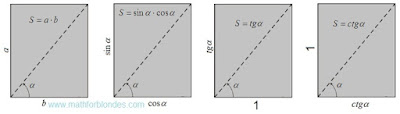Multiplication of the parties of a rectangle

For transition from the area of a rectangle to the simple area it is necessary to divide the square into itself. In this case two options of algebraic transformations are possible. It is necessary to highlight that the recommendation of mathematicians about reduction of identical quantities in numerator and a denominator of fraction is consciously controlled for identification of quantity of the received result. The first option of transformations looks as follows.First option of the unit area

The tangent and cotangent are connected by the inverse proportion. The parties of a rectangle can change somehow, from infinitely big quantity to infinitesimal. If change of the parties is carried out with keeping of the inverse proportion, then the area of a rectangle will remain invariable.

The second option of transformations is presented in an algebraic form and in physical units of measure.Second option of the unit area

This transformation shows that as a result of multiplication of two different units the third unit turns out. Multiplication is an interaction of two perpendicular units of measure as a result of which the new unit of measure turns out. As any quantity is result of multiplication of number and a unit of measure, under figure "one" can the number, a unit of measure or result of their interaction is meant.

Perpendicular units of measure are not connected among themselves in any way and can have the arbitrariest scale. The scale of units of measure of factors scales work units of measure, but does not influence a process essence – multiplication leads to high-quality change of initial units of measure.

The area is a result of interaction of two perpendicular measurements of length. If to multiplication inches by meters or millimeters on meters, then the area will be expressed in inches on meter or millimeters on meter. Traditionally, the area can be expressed in identical units of measure of length and width. In practice frames with different scales on a vertical and a horizontal are often used.

At determination of the area of a square the size of the party can be built in the second degree. But it does not mean at all that the party of a square is multiplied by itself. The area can still be determined only by multiplication of length to width, just at a square they have identical numerical values. We never multiply rectangle length by length or width on width because the result of such actions does not make sense. The involution is a multiplication of the different perpendicular quantities having identical numerical values and units of measure.

Algebraic transformations of multiplication of two to a square can be written down so:Algebraic transformations

If to consider that quantity a at a quadrating is multiplied by itself, then the inverse transformation of a square in work of two sums will be impossible. As it is possible to execute a similitude, is shown in the partition Decomposition of sume below.

Diagonal of a rectangle and angle between the diagonal and the party are padding characteristics of the interacting quantities.

At the following lesson we will consider
Examples of multiplication